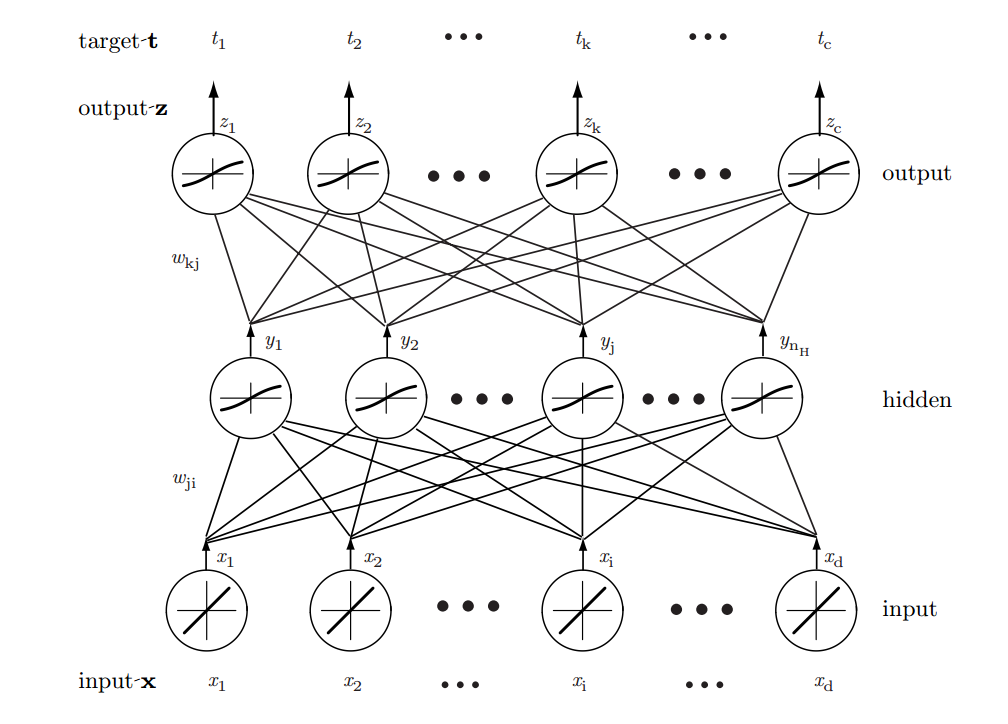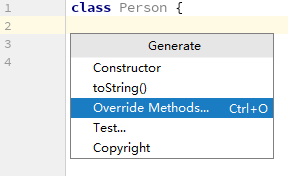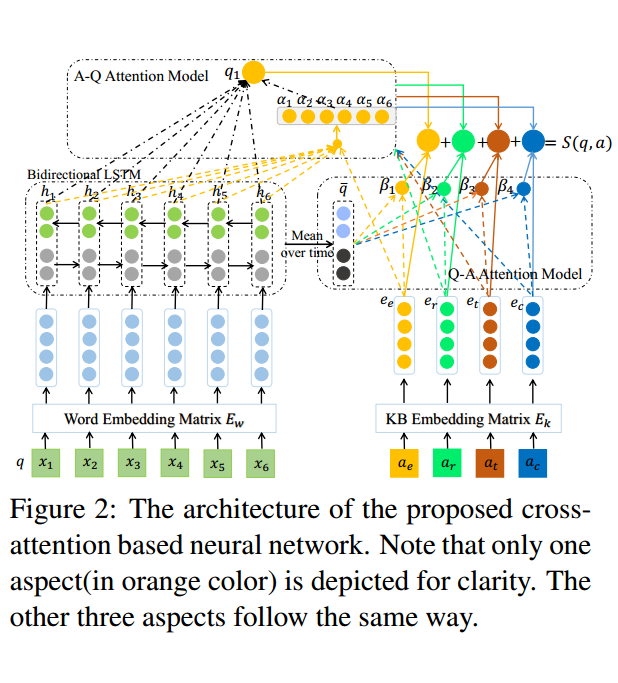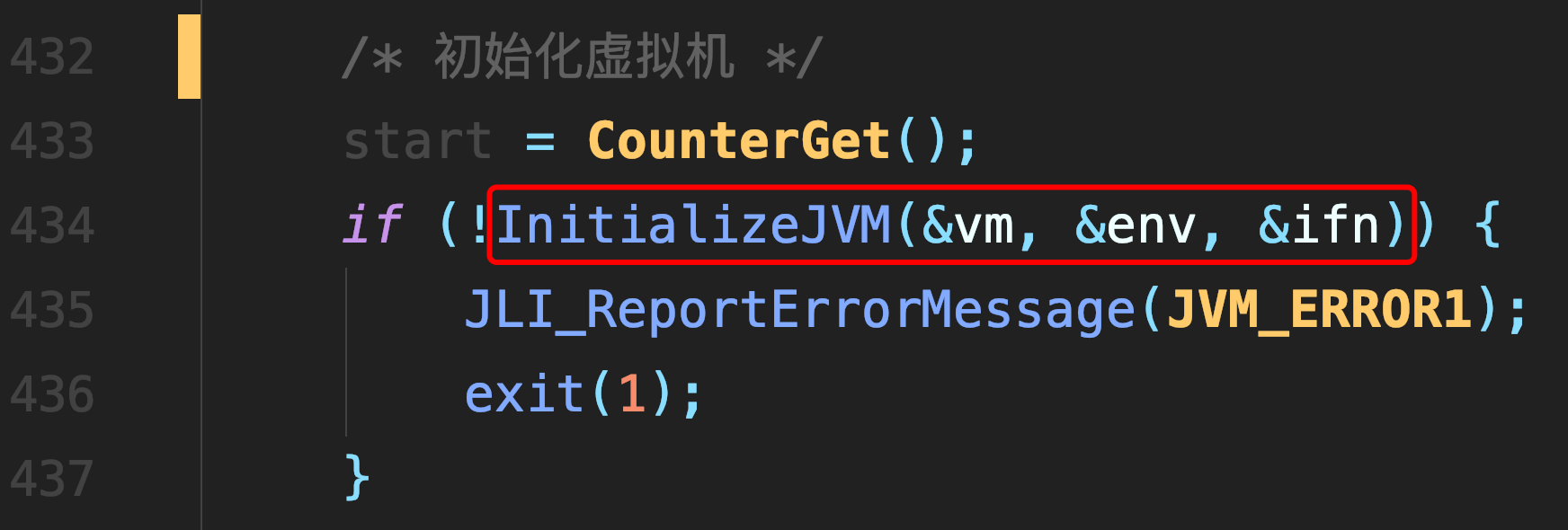# 反向传播算法

### 反向传播算法

• 下图是一个三层的神经网络，分别为输入层，隐含层，输出层。反向传播算法基于此图推导下面是一些变量的含义

$x_1, x_2,x_3...x_n$分别是输入单个样本向量的各个维的值

$y_1, y_2,....y_{nH}$分别是隐含层各个单元的输出值

$z_1,z_2,...z_c$分别是输出向量各个维的值。如果是分类问题，值最大的那维，就对应相应的预测类别

$t_1,t_2,...t_c$是相应输出向量各个维的真实值，也就是目标值。

$w_{kj}$代表第j个隐含单元到第k个输出单元的权值

$w_{ji}$代表第i个输入单元到第j个隐含单元的权值

$net_k=\sum\limits_{j=1}^{n_H}y_jw_{kj}+w_{k0}$代表所有隐含层单元对一个输出单元的净激活(没有加激活函数)

• ##### 训练误差

$J(\textbf{w})=\frac{1}{2}\sum\limits_{k=1}^{c}(t_k-z_k)^2=\frac{1}{2}||\textbf{t-z}||$

很容易理解，这就是一个均方差，加了 $\frac{1}{2}$是为了后面的计算。

• ##### 梯度下降参考链接

$\Delta\textbf{w}=-\eta\frac{\partial J}{\partial \textbf{w}} \\ \Delta{w_{pq}}=-\eta\frac{\partial J}{\partial {w_{pq}}} \\$

沿梯度的反方向调整参数，尽量减少训练误差。第二个公式是误差对单个权值的偏导

• ##### 求隐含层到输出层权值偏导 ${\partial \textbf J}/{\partial w_{kj}}$(使用链式求导法则)

$\frac{\partial\textbf{J}}{\partial w_{kj}} =\frac{\partial\textbf{J}}{\partial net_{k}}\frac{\partial{net_k}}{\partial w_{kj}}$

定义 $\delta_k?$为输出单元 $k?$的敏感度
$\delta_k=-\partial\textbf{J}/\ \partial net_k \\ =-\frac{\partial\textbf{J}}{\partial z_{k}}\frac{\partial z_k}{\partial net_{k}} \\ =(t_k-z_k)f^{'}(net_k)$
${\partial{net_k}}/{\partial w_{kj}}=y_j$

$\frac{\partial\textbf{J}}{\partial w_{kj}}=\eta\delta_ky_j=\eta(t_k-z_k)f^{'}(net_k)y_j$

• ##### 求输入层到隐含层权值偏导 $\partial \textbf J /{\partial w_{ji}}$

$\frac{\partial\textbf{J}}{\partial w_{ji}} =\frac{\partial\textbf{J}}{\partial y_{j}}\frac{\partial{y_j}}{\partial net_{j}}\frac{\partial{net_j}}{\partial w_{ji}} \\ \frac{\partial\textbf{J}}{\partial y_{j}} =\frac{\partial\textbf{J}}{\partial z_{k}}\frac{\partial{z_k}}{\partial y_{j}} \\ =-\sum\limits_{k=1}^{c}(t_k-z_k)\frac{\partial z_k}{\partial y_j} \\ =-\sum\limits_{k=1}^{c}(t_k-z_k)\frac{\partial z_k}{\partial net_k}\frac{\partial net_k}{\partial y_j} \\ =-\sum\limits_{k=1}^{c}(t_k-z_k)f^{'}(net_k)w_{kj}$

定义 $\delta_j$为隐单元 $j$的敏感度
$\delta_j=f^{'}(net_j)\sum\limits_{k=1}^{c}w_{kj}\delta_k$

$\frac{\partial\textbf{J}}{\partial w_{ji}}=\eta x_i \delta_j =\eta[\sum\limits_{k=1}^{c}w_{kj}\delta_k]f^{'}(net_j)x_i$
一个隐单元的敏感度是各输出单元敏感度的加权和，权重为 $w_{kj}$,然后与 $f^{'}(net_j)$相称乘。

##### 热门推荐
•浏览(654)
•浏览(563)
•浏览(547)
•浏览(480)
•浏览(424)
•浏览(419)
•浏览(381)
•浏览(366)
•浏览(362)
•浏览(344)